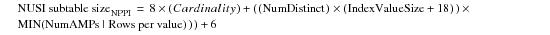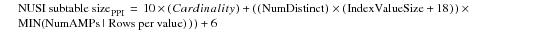# 15.00 - NUSI Sizing Equation - Teradata Database

prodname
vrm_release
15.00
category
User Guide
featnum
B035-1094-015K

### NUSI Sizing Equation

The following parameter definitions are used with this equation.

 Parameter Definition Cardinality x 8 Cardinality x 10 Each base table rowID is stored in a NUSI subtable. For an nonpartitioned primary index table, the row ID is 8 bytes long. For a PPI table, the row ID is 10 bytes long. This means that you must use the Cardinality * 8 factor for NUSI subtables for nonpartitioned primary index base tables and the Cardinality * 10 factor for NUSI subtables for PPI base tables. NumDistinct The value is an estimate of the number of distinct NUSI subtable values and is based on each NUSI subtable having at least one index row per AMP for each distinct index value of a base table row stored on that AMP. IndexValueSize The number of index data bytes. NUSI Row Overhead Sum of the following factors. Row headers and trailers NUSI row rowID Spare byte Presence octets       = 18 bytes MIN (NumAmps | Rows per value) The lesser of the two parameters. 6 The number of bytes consumed by the 3 VARCHAR Offset fields that follow the Additional Overhead field.

If fallback is defined for the base table, then double the calculated result.

#### NUSI Sizing Equation for Nonpartitioned Primary Index Base Table#### NUSI Sizing Equation for PPI Base Table